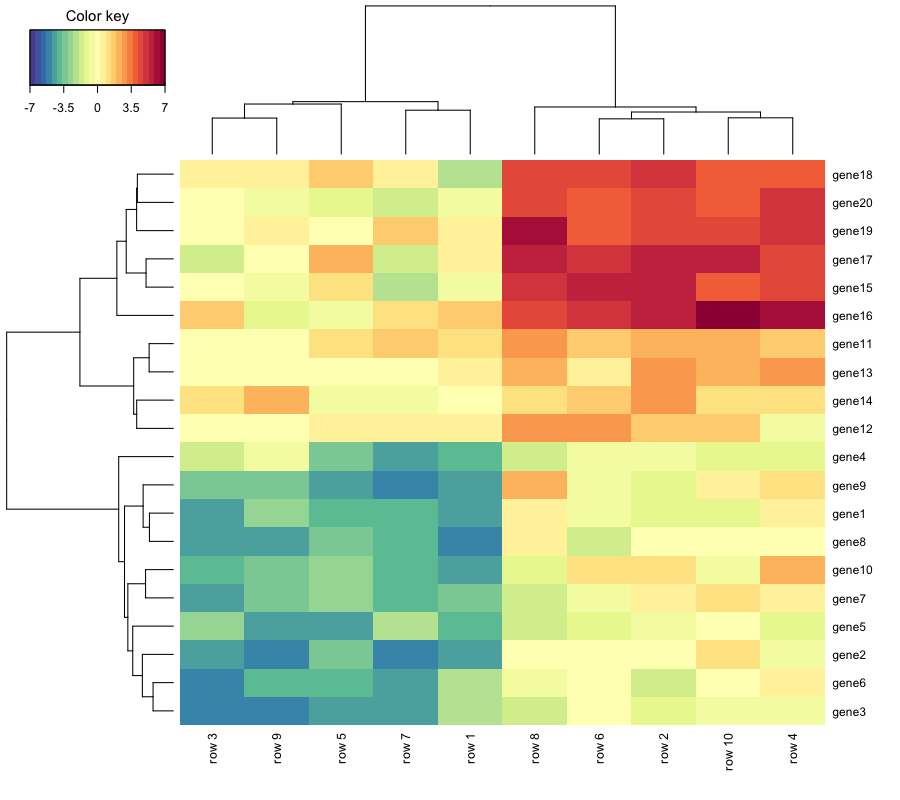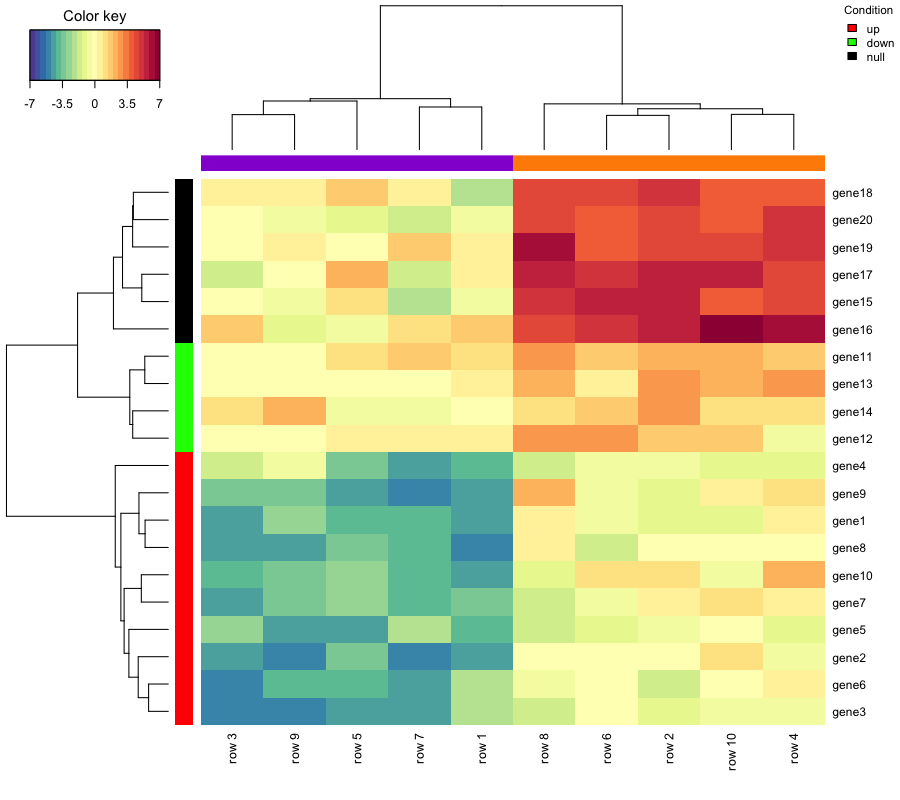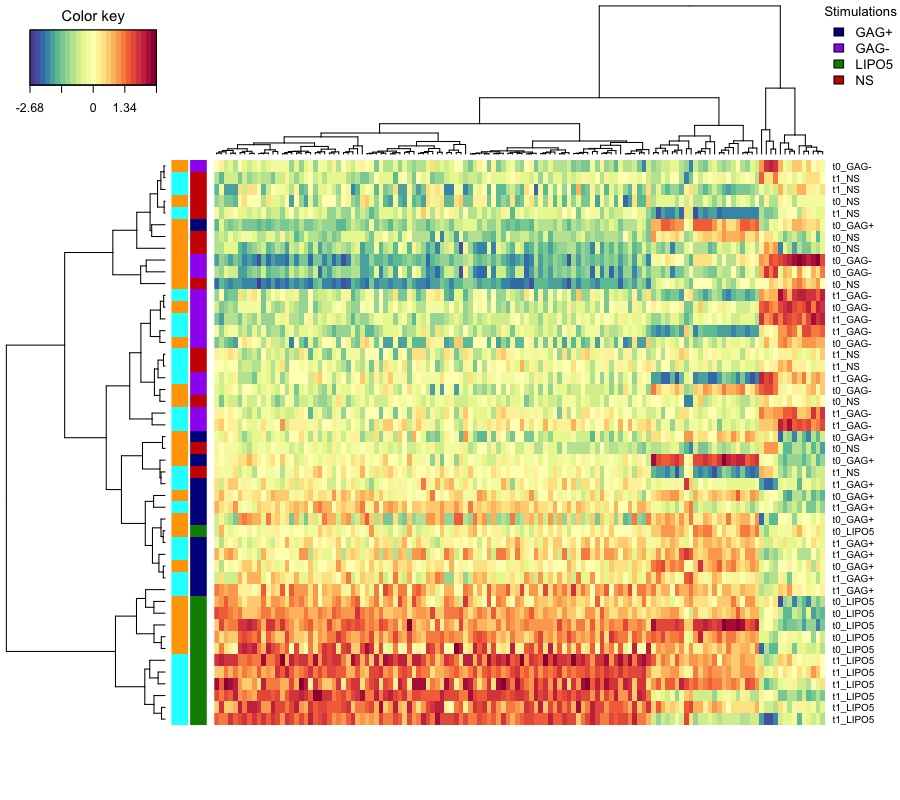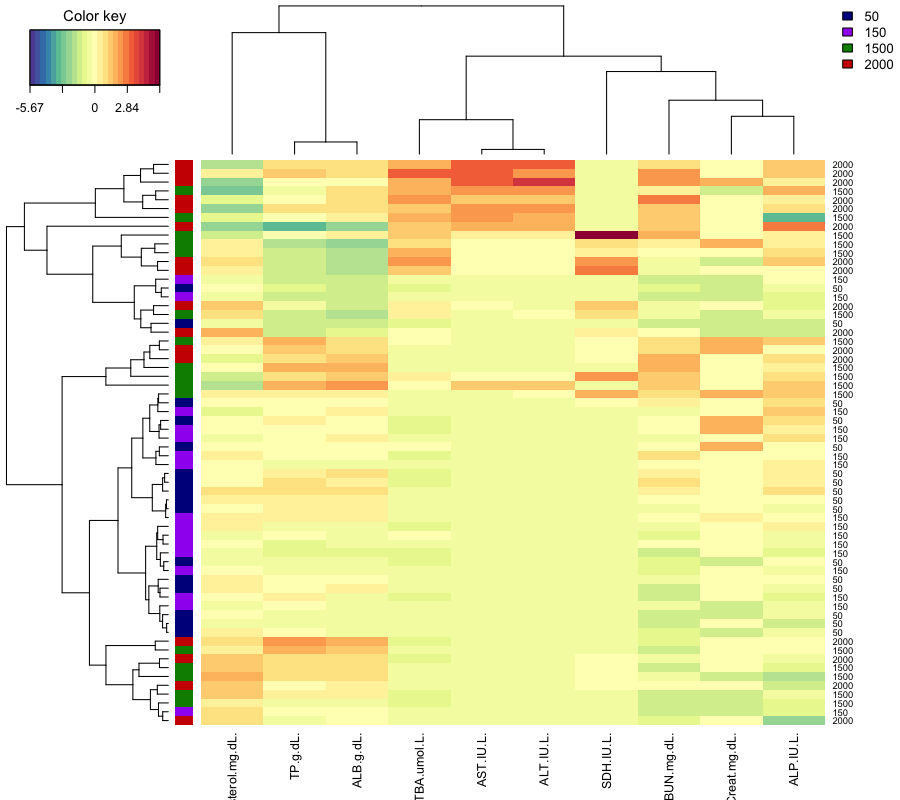# Clustered Image Maps

…is based on a hierarchical clustering simultaneously applied on the rows and the columns of a real-valued similarity matrix. This similarity matrix is obtained via the results of rCCA or (s)PLS. The matrix is graphically represented as a 2-dimensional colored image, where each entry of the matrix is colored on the basis of its value, and where the rows and columns are reordered according to a hierarchical clustering. Dendrograms resulting from the clustering are added to the left (or right) side and to the top (or bottom) of the image.

# Usage in mixOmics

CIM can be obtained in mixOmics via the function cim() as displayed below:

library(mixOmics)

set.seed(123)
mat <- matrix(rnorm(200), 20, 10)
mat[ 1:10, seq(1, 10, 2)] <- mat[ 1:10, seq(1, 10, 2)] - 4
mat[11:20, seq(2, 10, 2)] <- mat[11:20, seq(2, 10, 2)] + 2
mat[15:20, seq(2, 10, 2)] <- mat[15:20, seq(2, 10, 2)] + 3
colnames(mat) <- paste("row", 1:10, sep = " ")
rownames(mat) <- paste("gene", 1:20, sep = "")
#test col.sideColors
cond=rep(c("cond 1","cond 2"),5)
cond.col <- c("cond 1" = "darkviolet", "cond 2" = "darkorange")
obj.cim=cim(mat)#test row.sideColors
ddr <- as.hclust(obj.cim$ddr) cl <- cutree(ddr, k = 3) gene.col <- c("up" = "red", "down" = "green", "null" = "black") cim(mat, col.sideColors = cond.col[cond],row.sideColors = gene.col[cl], legend=list( legend = names(gene.col), col = gene.col,title = "Condition", cex = 0.7))data(vac18.simulated) X <- vac18.simulated$genes
Y <- vac18.simulated$stimulation design <- data.frame(samp = vac18.simulated$sample,
stim = vac18.simulated$stimulation, time = vac18.simulated$time)

res.2level <- splsda(X, Y, ncomp = 2, keepX = c(120, 10))

stim.col <- c("darkblue", "purple", "green4","red3")
stim.col <- stim.col[as.numeric(design$stim)] time.col <- c("orange", "cyan")[as.numeric(design$time)]

cim(res.2level, row.sideColors = cbind(stim.col, time.col),
row.names = paste(design$time, design$stim, sep = "_"),
col.names = FALSE,
legend=list(title="Stimulations"))data(liver.toxicity)

repeat.indiv <- c(1, 2, 1, 2, 1, 2, 1, 2, 3, 3, 4, 3, 4, 3, 4, 4, 5, 6, 5, 5,
6, 5, 6, 7, 7, 8, 6, 7, 8, 7, 8, 8, 9, 10, 9, 10, 11, 9, 9,
10, 11, 12, 12, 10, 11, 12, 11, 12, 13, 14, 13, 14, 13, 14,
13, 14, 15, 16, 15, 16, 15, 16, 15, 16)

design <- data.frame(sample = vac18$sample) res.spls.1level <- spls(X = liver.toxicity$gene,
Y=liver.toxicity$clinic, ncomp = 3, keepX = c(50, 50, 50), keepY = c(5,5,5), mode = "canonical") col <- c("darkblue", "purple", "green4","red3") cim(res.spls.1level, mapping="Y", row.names=liver.toxicity$treatment[,3],
row.sideColors = col[factor(liver.toxicity$treatment[,3])], legend=list(legend=unique(liver.toxicity$treatment[,3]),
col=col))# References

González I., Lê Cao K.-A., Davis, M.D. and Déjean S. (2013) Insightful graphical outputs to explore relationships between two ‘omics’ data sets. BioData Mining 5:19